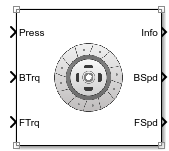# Disc Clutch

Idealized disc clutch coupler

• Library:
• Powertrain Blockset / Drivetrain / Couplings

•## Description

The Disc Clutch block implements an idealized disc clutch coupler. The block couples the rotary input and output shafts through an idealized friction model. To determine the output torque, the block uses friction parameters, relative slip velocity, and applied input pressure.

In fuel economy and powertrain efficiency studies, you can use the Disc Clutch block to model the mechanical power transfer between common driveline elements such as transmissions, engines, and differentials.

To approximate the torque response, the Disc Clutch block implements friction and dynamic models that depend on the clutch lockup condition. The block determines the locked or unlocked condition based on an idealized dry clutch friction model. This table summarizes the logic the block uses to determine the clutch condition.

Clutch ConditionWhen
Unlocked$\begin{array}{l}{\omega }_{i}\ne {\omega }_{o}\\ \text{or}\\ {T}_{fmax}<|\frac{{J}_{o}{T}_{i}-\left({J}_{o}{b}_{i}-{J}_{i}{b}_{o}\right){\omega }_{i/o}}{{J}_{o}+{J}_{i}}|\end{array}$
Locked$\begin{array}{l}{\omega }_{i}={\omega }_{o}\\ \text{and}\\ {T}_{fmax}<|{T}_{i}-\frac{{J}_{i}\left({b}_{i}+{b}_{o}\right){\omega }_{i}}{{J}_{o}+{J}_{i}}+{b}_{o}{\omega }_{i}|\end{array}$

This table summarizes the friction and dynamic models that the block uses for locked or unlocked clutch conditions.

Clutch ConditionFriction ModelDynamic Model
Unlocked$\begin{array}{l}{T}_{fmax}={T}_{k}\\ \text{where,}\\ {T}_{k}={N}_{disc}{P}_{c}{A}_{eff}{R}_{eff}{\mu }_{k}\mathrm{tanh}\left[4\left({\omega }_{i}-{\omega }_{o}\right)\right]\\ {R}_{eff}=\frac{2\left({R}_{o}{}^{3}-{R}_{i}{}^{3}\right)}{3\left({R}_{o}{}^{2}-{R}_{i}{}^{2}\right)}\end{array}$ and${P}_{c}=\mathrm{max}\left({P}_{c}-{P}_{eng},0\right)$$\begin{array}{l}{\stackrel{˙}{\omega }}_{i}{J}_{i}={T}_{i}-{T}_{f}-{\omega }_{i}{b}_{i}\\ {\stackrel{˙}{\omega }}_{o}{J}_{o}={T}_{f}+{T}_{o}-{\omega }_{o}{b}_{o}\end{array}$
Locked$\begin{array}{l}{T}_{fmax}={T}_{s}\\ \text{where,}\\ {T}_{s}={N}_{disc}{P}_{c}{A}_{eff}{R}_{eff}{\mu }_{s}\\ {R}_{eff}=\frac{2\left({R}_{o}{}^{3}-{R}_{i}{}^{3}\right)}{3\left({R}_{o}{}^{2}-{R}_{i}{}^{2}\right)}\end{array}$$\begin{array}{l}{\stackrel{˙}{\omega }}_{i}\left({J}_{o}+{J}_{i}\right)={T}_{o}-{\omega }_{i}\left({b}_{i}+{b}_{o}\right)+{T}_{i}\\ {\omega }_{i}={\omega }_{o}\end{array}$

### Power Accounting

For the power accounting, the block implements these equations.

Bus Signal DescriptionEquations

`PwrInfo`

`PwrTrnsfrd` — Power transferred between blocks

• Positive signals indicate flow into block

• Negative signals indicate flow out of block

`PwrBase`

Applied base power

${\omega }_{i}{T}_{i}$

`PwrFlwr`

Applied follower output power

${\omega }_{o}{T}_{o}$

`PwrNotTrnsfrd` — Power crossing the block boundary, but not transferred

• Positive signals indicate an input

• Negative signals indicate a loss

`PwrDampLoss`

Damping power loss

`PwrCltchSlipLoss`

Clutch slip power loss

$-{T}_{k}\left({\omega }_{i}-{\omega }_{o}\right)$

`PwrStored` — Stored energy rate of change

• Positive signals indicate an increase

• Negative signals indicate a decrease

`PwrStoredBase`

Rate change in base rotational kinetic energy

${\stackrel{˙}{\omega }}_{i}{\omega }_{i}{J}_{i}$

`PwrStoredFlwr`

Rate change in follower rotational kinetic energy

${\stackrel{˙}{\omega }}_{o}{\omega }_{o}{J}_{o}$

The equations use these variables.

 ωi Input shaft angular speed ωo Output shaft angular speed bi Input shaft viscous damping bo Output shaft viscous damping Ji Input shaft moment of inertia Jo Output shaft moment of inertia Tf Frictional torque Ti Net input torque Tk Kinetic frictional torque To Net output torque Ts Static frictional torque Tfmax Maximum frictional torque before slipping Pc Applied clutch pressure Peng Engagement pressure Aeff Effective area Ndisc Number of frictional discs Reff Effective clutch radius Ro Annular disk outer radius Ri Annular disk inner radius Re Effective tire radius while under load and for a given pressure μs Coefficient of static friction μk Coefficient of kinetic friction

## Ports

### Input

expand all

Base gear input torque, Pc, in N·m^2.

Applied input torque, Ti, typically from the engine crankshaft or dual mass flywheel damper, in N·m.

#### Dependencies

To create this port, for Port Configuration, select `Simulink`.

Applied load torque, To, typically from the differential or drive shaft, in N·m.

#### Dependencies

To create this port, for Port Configuration, select `Simulink`.

Applied drive shaft angular speed, ωi, in rad/s. Applied drive shaft torque, Ti, in N·m.

#### Dependencies

To create this port, for Port Configuration, select `Two-way connection`.

### Output

expand all

Bus signal containing these block calculations.

SignalDescriptionUnits
`Base``BTrq`

Applied input torque, typically from the engine crankshaft or dual mass flywheel damper

N·m

`BSpd`

Applied drive shaft angular speed input

`Flwr``FTrq`

Applied load torque, typically from the differential

N·m

`FSpd`

Drive shaft angular speed output

`Cltch``CltchForce`

Applied clutch force

N

`CltchLocked`

Clutch lock status

NA

`CltchSpdRatio`

Clutch speed ratio

NA

`CltchEta`

Clutch power transmission efficiency

NA

`PwrInfo`

`PwrTrnsfrd`

`PwrBase`

Applied base power

W
`PwrFlwr`

Applied follower output power

W

`PwrNotTrnsfrd`

`PwrDampLoss`

Damping power loss

W
`PwrCltchSlipLoss`

Clutch slip power loss

W

`PwrStored`

`PwrStoredBase`

Rate change in base rotational kinetic energy

W
`PwrStoredFlwr`

Rate change in follower rotational kinetic energy

W

Applied drive shaft angular speed input, ωi, in rad/s.

#### Dependencies

To create this port, for Port Configuration, select `Simulink`.

Drive shaft angular speed output, ωo, in rad/s.

#### Dependencies

To create this port, for Port Configuration, select `Simulink`.

Output drive shaft angular speed, ωoi, in rad/s. Output drive shaft torque, To, in N·m.

#### Dependencies

To create this port, for Port Configuration, select `Two-way connection`.

## Parameters

expand all

Block Options

Specify the port configuration.

#### Dependencies

Specifying `Simulink` creates these ports:

• `BSpd`

• `FSpd`

• `BTrq`

• `FTrq`

Specifying `Two-way connection` creates these ports:

• `B`

• `F`

Clutch force equivalent net radius, in m.

Number of disks, dimensionless.

Effective applied pressure area, in m^2.

Pressure to engage clutch, in Pa.

Input shaft inertia, in kg·m^2.

Output shaft inertia, in kg·m^2.

Kinetic friction coefficient, dimensionless.

Static friction coefficient, dimensionless.

Input shaft viscous damping, in N·m· s/rad.

Output shaft viscous damping, in N·m· s/rad.

Input shaft initial velocity, in rad/s.

Input shaft initial velocity, in rad/s.

Clutch actuation time constant, in s.

Select to lock clutch initially.

## Extended Capabilities

### C/C++ Code GenerationGenerate C and C++ code using Simulink® Coder™.